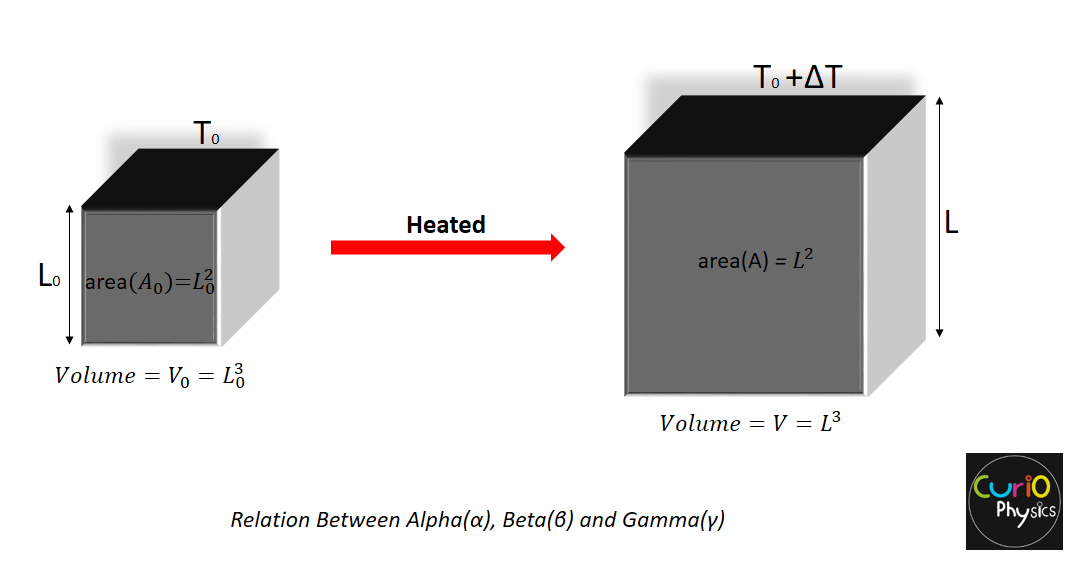# Relation Between alpha beta and gamma

### Relation Between alpha beta and gamma

We have already studied about alpha beta and gamma in thermal expansion. In this article we are going to discuss that what is the Relation Between alpha beta and gamma in physics?

Suppose that there is a cube of some material of side L having :-

• Coefficient of linear expansion (α)
• Coefficient of areal expansion (β)   and
• Coefficient of volumetric expansion (γ)

Let the cube be heated by a small range of temperature ΔT, due to which its dimensions changes.New side length                            L = L0 (1 + αΔT )     ……….(1)

New surface area of one face     A = A0 (1 + βΔT )    ……….(2)

New volume                                  V = V0 (1 + γΔT )    ……….(3)

Now, let us derive the relation Between alpha beta and gamma.

New surface area of one face can also be written as

A0+ΔA  =  (L)2 = (L0 + ΔL)2

A0+βA0ΔT  = (L0 + αL0ΔT)2

A0 (1 + βΔT) = (L0)2(1 + αΔT)2

A0 (1 + βΔT) = (L0)2(1 + αΔT)2

because A0 = (L0)2

⇒  1 + βΔT = (1 + αΔT)2

⇒  1 + βΔT = 1 + α2 ΔT2 + 2αΔT

⇒    βΔT  = α2 ΔT2 + 2αΔT

The value of α is of the order of 10-5 so its higher powers can be neglected i.e., α2 ΔT2 ≈ 0

This gives us

βΔT  =  2αΔT

βΔT  =  2αΔT

⇒   β = 2α  or  α = β/2     ……….(4)

Again new volume V can be written as

V0 + ΔV = (L)3 = (L0 + ΔL)3

V0 + γV0ΔT  = (L0 + αL0ΔT)3

V0(1 + γΔT)  = (L0)3(1 + αΔT)3

because V0 = (L0)3

V0(1 + γΔT)  = (L0)3(1 + αΔT)3

1 + γΔT  = (1 + αΔT)3

1 + γΔT  = 1 + α3ΔT3 +3αΔT + 3α2 ΔT2

again neglecting the terms containing higher powers of α, i.e., α3ΔT3 ≈ 0 and 3α2 ΔT2 ≈ 0 , we get

1 + γΔT = 1 + 3αΔT

⇒     γΔT = 3αΔT

⇒  γ = 3α  or  α = γ/3     ……….(5)

From equations (4) and (5),

 α = β/2  =  γ/3

or

 6α = 3β  =  2γ

This is the required relation between alpha beta and gamma.

 Also α : β : γ = 1 : 2 : 3